#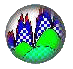Interactive Real Analysis - part of MathCS.org

Next | Previous | Glossary | Map | Discussion

## 7.3. Measures

The Riemann integral is certainly useful (and complicated) enough, but it does have a few limitations and oddities:
 Examples 7.3.1:Oddities of Riemann Integral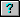What happens when you change the value of a Riemann integrable function at a single point?Is it true that a function that is constant except at countably many points is Riemann integrable?What is the difference between Riemann integrable functions and bounded continuous functions?Can you take a Riemann integral over anything else but an interval?Could you define a Riemann integral of a function whose domain is not R?There is a sequence of Riemann integrable functions fn that converges to a function f that is not Riemann integrable.There is a sequence of Riemann integrable functions fn that converges to another Riemann integrable function f but the corresponding sequence of Riemann integrals of fn does not converge to the integral of f.

We therefore want to define another concept of integration that is more general than the Riemann integral, yet retains the "good" properties of that integral.

One of the limitations of the Riemann integral is that it is based on the concept of an "interval", or rather on the length of subintervals [xj-1, xj]. We therefore need to find a generalization of the "length" concept of a set in the real line. That new "length" concept, which we will call "measure", should satisfy two key conditions:

1. The new "measure" concept should be applicable to intervals, unions of intervals, and to more general sets (such as a Cantor set). Ideally, it should be defined for all sets.
2. The new "measure" concept should share as many properties as possible with the standard length of an interval, such as:
• the 'measure' of a set should be non-negative
• the 'measure' of an interval should be the length of that interval
• the 'measure' of countably many disjoint sets should be the sum of the 'measures' of the individual sets
To define what will eventually be called Lebesgue Measure, we follow a two-stage strategy:
Stage One:
We will define a concept extending length that is defined for all sets (to satisfy condition 1 above)
Stage Two:
We will modify that concept so that it looks as close as possible to the standard length concept (to satisfy condition 2 above)
The stage-one concept is called outer measure, defined as follows:
 Definition 7.3.2: Outer Measure If A is any subset of R, define the (Lebesgue) outer measure of A as: m*(A) = inf {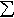l(An) } where the infimum is taken over all countable collections of open intervals An such that A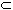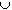An and l(An) is the standard length of the interval An.

Note that it is possible to define other outer measures without using the concept of length of intervals, so the above outer measures is but one of many. In fact, this particular outer measure is called the Lebesque outer measure, but we will not talk about any other outer measure in this chapter, so whenever we say "outer measure" we really mean "Lebesque outer measure".

 Examples 7.3.3: Outer Measure of IntervalsFind the outer measure of the empty set O, and prove that m*(A)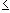m*(B) for all AB.Find the outer measure of a closed interval [a, b]Find the outer measure of an open interval (a, b)Find the outer measure of an infinite intervalFind the outer measure of the set A of all rational numbers in [0, 1]. Also show that for any finite collection of intervals covering A we have that the sum of their lengths is greater or equal to 1.
Outer measure is defined for all sets in R and has some of the properties we wanted our concept of measure to have.
 Proposition 7.3.4: Properties of Outer Measure Outer measure has the following properties: Outer measure m* is a non-negative set function whose domain is P(R), i.e. the power set of R. The outer measure of an interval is its length. Outer measure is subadditive, i.e. m*(AB)m*(A) + m*(B) Outer measure is countably subadditive, i.e. if { An } is a countable collection of sets, then m*(An)m*(An)

Since outer measure is defined for all sets in R, our first key condition above is satisfied. But outer measure is not quite similar to a length, because it is only subadditive ( m*(AB)m*(A) + m*(B) ), not additive ( m*(AB) = m*(A) + m*(B) for disjoint sets A and B). Therefore one of the requirements of the second key condition is not satisfied.

 Examples 7.3.5: Properties of Outer MeasureShow that the outer measure of a single point is 0, and the outer measure of a countable set is also 0.The set [0, 1] is not countable.Outer measure is subadditive, but not additive.Define a function f on the set X = {a, b, c} by setting f(O) = 0, f(X) = 2, and f(A) = 1 for any other subset A of X. Could this function be called an outer measure, i.e. is it a non-negative set function whose domain is P(R) and which is subadditive? Is it additive?

To make our outer measure additive, something has to give. We decide to restrict the domain to gain countable additivity, which will result in our stage-two definition.

Actually, there are several ways to restrict an outer measure. A somewhat intuitive way, perhaps, is to define an inner measure m* that is similar to the outer measure but involves a sup over suitable sets. Then sets for which m*(A) = m*(A) are called measurable and the restriction of the outer (or inner) measure to the measurable sets is called a measure. Another idea is to define a set E as measurable if it is "almost" an open set, in the sense that for every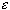> 0 there exists an open set UR such that m*(U \ E). This is an idea known as one of Littlewood's three principles, which is helpful enough, but we will choose yet another approach due to Caratheodory that is (hopefully) less abstract and (somewhat) easier to work with.

 Definition 7.3.6: Measurable Sets and Lebesgue Measure A set E is (Lebesque) measurable if for every set A we have that m*(A) = m*(A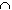E) + m*(Acomp(E)) If E is measurable, the non-negative number m(E) = m*(E) is the (Lebesgue) measure of the set E.
Since outer measure is subadditive, we have
m*(A) = m*( (AE)(Acomp(E)) )m*(AE) + m*(Acomp(E))
Therefore, to show that a set E is measurable it is sufficient to prove that
m*(A)m*(AE) + m*(Acomp(E))
The motivation for this definition is that it ensures that for two disjoint measurable sets E and F we have that
m(EF) = m(E) + m(F)
as we will show later, i.e. measure is additive.
 Examples 7.3.7: Measurable SetsShow that the empty set, the set R, and the complement of a measurable set are all measurable.Show that every set with outer measure 0 is Lebesgue measurable.Show that the union of two measurable sets is measurable.Show that the intersection of two measurable sets is measurable.Show that the interval (a,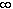) is measurable.

The next result shows that a measure has all of the properties of a length: the measure of an interval is its length, and the measure of countably many disjoint sets it is additive. But, as we mentioned before, the price we have to pay for that is that it is no longer defined for all sets.

 Theorem 7.3.8: Properties of Lebesgue measure All intervals are measurable and the measure of an interval is its length All open and closed sets are measurable The union and intersection of a finite or countable number of measurable sets is again measurable If A is measurable and A is the union of countable number of measurable sets An, then m(A)m(An) If A is measurable and A is the union of countable number of disjoint measurable sets An, then m(A) =m(An)

Sometimes we refer to the collection of all open sets, closed sets, and sets that can be derived from them as Borel sets. Its significance for our discussion is that all Borel sets are measurable. So, instead of listing open sets, closed sets, unions of them, intersections of them, etc. we refer to them collectively as Borel sets. However, it is important to note that not every Borel set is a union or intersection of open/closed sets, and unions/intersections of those, etc., so that the Borel sets are really something new. But these details would lead us to far afield.

 Theorem 7.3.9: Borel sets are measurable The collection of Borel sets is the smallest sigma-algebra which contains all of the open sets. Every Borel set, in particular every open and closed set, is measurable.

After all this theory it is time to consider some easier examples, including the measure of some well-known sets that are not intervals (whose measure, as we know by now, are their length).

 Examples 7.3.10: Properties of MeasureShow that for any two sets A and B we have that m(A - B) = m(A) - m(AB) . What if BA?What is the measure of the set Q of all rational numbers and the set I of all irrational numbers inside [0, 1].Find the measure of the Cantor middle-third set (if it measurable).

Outer measure is defined for all sets, and according to the above list of properties, most "common" sets such as intervals, closed or open sets, unions and intersections of measurable sets are all measurable. But we have to pay for the property that measure is (countably) additive with the fact that not every set is measurable, as we will see shortly. First, let's take a quick look into sets that arise in conjunction with functions? First, let's establish some equivalency conditions.

 Proposition 7.3.11: Equivalent Measurable Sets Let f be a real-valued function with a measurable set as domain. Then the following are quivalent: the set { f(x)a } is measurable for all real numbers a the set { f(x) < a } is measurable for all real numbers a the set { f(x)a } is measurable for all real numbers a the set { f(x) > a } is measurable for all real numbers a

Note that this does not say that each or any of these sets are indeed measurable. It does say that if any of these four sets are measurable, then the remaining ones are also measurable. An easy case in which one of these sets is clearly measurable is described next.

 Proposition 7.3.12: Inverse Images are Measurable If f is a continuous function with a measurable set as domain, then the sets f -1(a, b), f -1(a, b], f -1[a, b), and f -1[a, b] are all measurable for any (extended) real numbers a < b.

So here we have further examples of sets that are measurable. But not all is roses, and you can't always have your cake and eat it, too: we do have to pay for the property that measure is (countably) additive with the fact that not every set is measurable.

 Proposition 7.3.13: Not all Sets are Measurable There are sets that are not (Lebesgue) measurable, i.e. not every set is (Lebesgue) measurable.

It is therefore true that not every set is measurable, but it is fair to say that most sets are.

To summarize, we introduced a new concept called measure in two stages, each of which had a typical "good news, bad news" property.

1. In the first stage we defined outer measure.
• good news: outer measure is defined for all sets.
• bad news: outer measure is not additive, i.e. it is not quite comparable to a length.
2. In the second stage we defined measure by restricting outer measure to the measurable sets.
• good news: measure is additive, i.e. it is a good generalization of length.
• bad news: measure is not defined for all sets
Before concluding this section, we want to prove one more result that will be important for the next section:
 Proposition 7.3.14: Monotone Sequences of Measurable Sets If { An } is a sequence of measurable sets that is decreasing, i.e. AjAj+1 for all j, and m(A1) is finite, then lim m(Aj) = m(Aj) If { An } is a sequence of measurable sets that is increasing in the sense that Aj+1Aj for all j, then lim m(Aj) = m(Aj)
 Examples 7.3.15: Monotone sequences of measurable setsFor decreasing sets we had to assume that m(A1) was finite. Show that without this assumption the statement in the previous proposition is false.Find the measure of the Cantor middle-fifth set, i.e. the set obtained by using a Cantor-set construction but removing the middle-fifth instead of the middle-third at each stage.